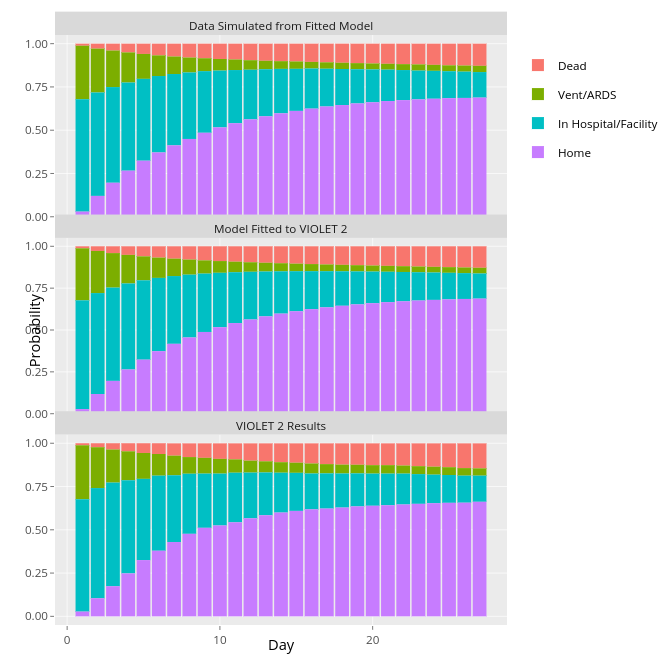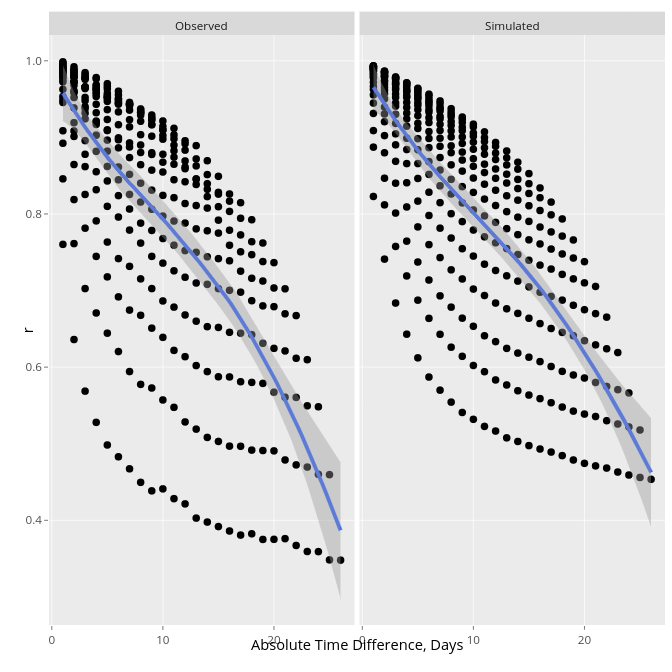Ann Arbor ASA Chapter  2022-03-22
FDA CDER Office of Biostatistics  Expanded 2023-06-21, 2023-07-11

## Background Questions

• How many have asked sponsors submitting $$t$$-tests and linear regression analysis to verify normality and parallelism (on $$\Phi^{-1}(F(y))$$ scale) assumptions (no relationship of an $$X$$ and $$\text{var}(Y|X)$$)?
• How many have asked sponsors submitting linear mixed model analysis to provide a variogram to demonstrate agreement between the assumed and actual within-patient correlation patterns?

## Background Questions, continued

• Do you know of anyone who has worried about the proportional hazards assumption and recommended the sponsor provide a logrank test instead?
• Do you know of anyone who has worried about the proportional odds assumption and recommended the sponsor provide a Wilcoxon test instead?

## Background Questions, continued

• Do you wonder why we have so many special cases in statistics that require seemingly different methods?
• time to first event
• recurrent events
• recurrent events with an absorbing state
• competing risks
• Wilcoxon, Kruskal-Wallis, logrank tests
• zero inflation adaptations of Poisson and negative binomial models

## Background Questions, continued

• Have you stopped to consider
• whether random effects are the first line of defense against within-patient correlation
• whether the emphasis given by random effects to patient-level outcome estimation is an important goal for treatment comparisons?

## Univariate Proportional Odds Model

• $$\Pr(Y \geq y | X) = \mathrm{expit}(\alpha_{y} + X\beta)$$
• $$Y$$-transformation invariant, does not use $$Y$$ spacings
• Handles arbitrarily heavy ties or continuous $$Y$$, bi-modality, …
• Direct competitor of the linear model

## PO Model, continued

• Wilcoxon-Mann-Whitney test: concordance probability $$c \approx \frac{OR^{0.66}}{1 + OR^{0.66}}$$ whether or not PO holds
• Kruskal-Wallis test: same; $$\beta$$ for $$X=j$$ vs. $$X=i$$ reflects $$c$$ for $$X$$ vs. $$Y$$ computed on observations with $$X \in \{i, j\}$$
• See fharrell.com/post/wpo

## Extensions to Allow Non-PO

• Partial PO model
• Constrained partial PO model
• analogous to unequal variances in linear models
• Peterson & Harrell, 1990

## Full Likelihood Extensions

• Random intercepts
• massive lack of fit for correlation structure
• implies there is interest in individual pt-level outcomes vs. group level (treatment level)
• absorbing states destroy the correlation pattern
• typically assumes that > 6 observations per patient do not increase power

## Full Likelihood Extensions, continued

• Random intercepts and slopes
• more flexible correlation structure but still may not fit
• too many parameters to estimate
• can’t have absorbing states
• Markov models
• most flexible, fastest, easiest to program
• trivial to implement with ML (until you want state occupancy probabilities)

## Full Likelihood Extensions, continued

• Markov models apply to all $$Y$$
• binary
• unordered categorical
• ordinal categorical
• ordinal continuous
• ordinal mixed continuous and categorical
• continuous
• left, right, and interval censored
• require unconditioning on previous $$Y$$ to get marginal distributions

## Full Likelihood Extensions, continued

• Other marginal models (Lee & Daniels, Schildcrout); direct $$\Pr($$state occupancy$$)$$
• don’t explicitly handle absorbing states

## First-Order Discrete Time Markov Proportional Odds Model

• Current state depends only on covariates, previous state, gap
• Let measurement times be $$t_{1}, t_{2}, \dots, t_{m}$$, and the measurement for a patient at time $$t$$ be denoted $$Y(t)$$ $\Pr(Y(t_{i}) \geq y | X, Y(t_{i-1})) =$ $\mathrm{expit}(\alpha_{y} + X\beta + g(Y(t_{i-1}), t_{i}, t_{i} - t_{i-1}))$

## Examples of Parametric Modeling Previous State in $$g$$:

• Linear in numeric codes for $$Y$$
• Single binary indicator for a specific state such as the lowest or highest one
• Discontinuous bi-linear relationship where there is a slope for in-hospital outcome severity, a separate slope for outpatient outcome severity, and an intercept jump at the transition from inpatient to outpatient (or vice versa).

## Most Important Effects to Include

• Previous state
• Flexible function of time $$t$$ since randomization
• No time effect $$\sim$$ constant hazard rate
• Non-proportional odds effects for $$t$$
• Mix of events can change over time, e.g., early ventilator use, late death
• $$t \times$$ previous state interaction
• Example: Hospitalized patients more stable over time = increasing effect of previous state

## Effects to Possibly Include, continued

• Flexible function of gap times (if gap times and absolute time are virtually collinear, one of these may be omitted)
• Interaction between previous state and gap time if gaps are very non-constant
• Interaction between time and treatment if treatment effect is delayed, etc.

## Markov PO Model: A Unified Approach

• Time to terminating event
• transition probability = discrete hazard rate
• OR $$\approx$$ HR when time intervals small
• easily handles time-dependent covariates, left-truncation
• Recurrent binary events
• Recurrent binary events + a terminal event

## Unified Approach, continued

• Competing risks
• death explicitly handled as a bad outcome
• easier to interpret than competing risk models
• Serial current status data
• events of different severities
• no need to judge whether an early heart attack is worse than a late death
• Missing data and interval-censored $$Y$$

## Unified Approach, continued

• Standard longitudinal continuous $$Y$$
• Longitudinal continuous or ordinal $$Y$$ interrupted by clinical events
• Easily handles multiple absorbing states

## Examples of Longitudinal Ordinal Outcomes

• censored at 3w: 000
• death at 2w: 01
• 0=at home 1=hospitalized 2=MI 3=dead
• hospitalized at 3w, rehosp at 7w, MI at 8w & stays in hosp, f/u ends at 10w: 0010001211

## Examples, continued

• 0-6 QOL excellent–poor, 7=MI 8=stroke 9=dead
• QOL varies, not assessed in 3w but pt event free, stroke at 8w, death 9w: 12[0-6]334589
• MI status unknown at 7w: 12[0-6]334[5,7]89
• Can make first 200 levels be a continuous response variable and the remaining values represent clinical event overrides

## Quality of Fit in a Critical Illness RCT

• VIOLET trial from the PETAL network, NHLBI
• Vitamin D in critically ill adults
• Daily assessments for 28d on 4-level ordinal $$Y$$: home, hospitalized, vent/ARDS, dead
• See here for details and code## Second-Order Fit

• Add random effects: negligible (indicates conditional independence)
• Variogram## Unconditioning on Previous States

• For equal time spacing:
$$\Pr(Y(t)=y | X) =$$
$$\sum_{j=1}^{k}\Pr(Y(t)=y | X, Y(t-1) = j) \times$$
$$\Pr(Y(t-1) = j | X)$$
• Use this recursively
• Yields a semiparametric unconditional (except for $$X$$) distribution of $$Y$$ at each $$t$$ (SOPs)
• soprobMarkovOrd* functions in the R Hmisc package make this easy for frequentist and Bayesian models

## Estimands

• Transition odds ratios (original parameters)
• Prior state and covariate-specific transition probabilities
• Covariate-specific SOPs
• $$\Pr($$stroke in week 4 or death in or before week 4$$)$$; $$\Pr($$stroke and alive$$)$$
• Time in state $$Y=y$$ (like RMST)
• Time in states $$Y \geq y$$ (e.g., mean time unwell)
• Differences in mean time in state between treatments

## Special Advantage of Bayesian Models and MCMC Posterior Sampling

• SOPs involve complex derived parameters for which frequentist CLs are very hard to derive
• Bayesian posterior distribution and uncertainty intervals derived from it are trivial to compute
• Example: 4,000 posterior draws from transition model’s basic parameters; compute 4,000 values of each derived parameter (SOP; mean time in state, etc.)

## Software

• Frequentist: R VGAM package
• Bayesian: R rmsb package

## Afterthoughts and Q&A

• See hbiostat.org/rmsc/long.html#modeling-within-subject-dependence for a table comparing repeated measures ANOVA, GEE, mixed effects, GLS, Markov, LOCF, and summary statistic approaches to longitudinal data
• Natural tendency for reviewers to be more accepting of familiar methods
• Many statisticians wrongly believe CLT protects finite sample size analyses

## Afterthoughts, continued

• Gaussian methods have far more assumptions that semiparametric models such as PO
• Mixed effects model for longitudinal data
• $$Y|X$$ is Gaussian
• constant variance (exact analogy of PO assumption)
• shape of $$X$$-relationships with $$E(Y|X)$$
• interactions among $$X$$ and with treatment

## Afterthoughts, continued

• Mixed effects model
• within-patient correlation of $$Y$$ at times $$a$$ and $$b$$ ($$a \neq b$$) is constant regardless of $$|a - b|$$
• unrealistic for long-duration follow-up
• failure to properly model how strongly repeated measurements are connected to each other $$\rightarrow$$ wrong SE(treatment effect), $$\alpha$$

## Assumptions of Longitudinal Models: Strategies

• Data from earlier trial or OS
• check that assumed model structure can reproduce
• first-order properties
• second-order properties (e.g., variogram)
• raw data
• Within-trial assumption checking

## Within-Trial Assumption Checking

• Secondary analyses
• Add random effects and estimate their variance
• Compare correlation vs. lag induced by model to that in raw data
• Add lag 2 (second order Markov)
• Impact of PO assumption for treatment
• Usual linearity and interaction assessments

## Q&A

• What assumptions are needed for longitudinal ordinal first-order Markov models?
• usual $$X$$-transformation and interaction assumptions
• distribution of $$Y$$ given all previous $$Y$$ is adequately captured by the previous $$Y$$
• distribution of $$Y$$ given previous $$Y$$ and $$X_1$$ is a simple shift of the shape given previous $$Y$$ and $$X_2$$ (PO assumption; analogy of equal variance assumption)

## Q&A, continued

• Note: distribution of $$Y$$ given previous $$Y$$ and $$X$$ can be any shape

## Q&A, continued

• Are these assumptions plausible?
• the data format used respects typical “current status” data generating processes
• excellent fits to previous datasets in similar disease/treatment situations
• direct modeling of correlation structure as covariates adds great flexibility and leads to simple diagnostics

## Plausibility of Assumptions, continued

• Compare with huge assumptions made in simple analyses
• MACE: patient dying a year after nonfatal MI $$\rightarrow$$ death completely ignored
• Nonfatal MI counted equally bad as death
• Recurrent hospitalizations are ignored
• PH for each endpoint may lead to non-PH for time to first endpoint

## Keep Things in Context

• Highly visible gross violations of assumptions about data
• Possible violation of assumptions about the data model

## Q&A, continued

• Does the output of these models produce clinically meaningful results, and how do clinical colleagues interpret such results?
• extensive experience in using these models with COVID-19 DSMBs
• daily stacked bar charts by treatment with covariate-adjusted outcome tendencies accepted immediately
• similar for posterior densities of covariate-specific differences between treatments in mean times in various states (esp. time with no or minimal symptoms)

## Q&A, continued

• Corresponding point estimates, e.g., “best guess of reduction in days unwell by treatment B is 0.5” (also include uncertainty interval)

## Q&A, ‘r cont’

• How to capture in a label
• Physicians and patients understand time more than risks
• Example for long-term CV study: treatment B provided an additional 0.5 years free of hospitalization, MI, or death
• As with any absolute quantity, the estimate will depend on baseline severity etc.
• $$\Pr(OR < 1) = 0.97$$: evidence for benefit; posterior probability is independent of baseline severity unless treatment interacts with a covariate

## Q&A, continued

• How to implement in a sequential design?
• Frequentist: simulate $$\alpha$$ based on look intentions
• Bayesian: trivial, since current posterior probability is self-contained